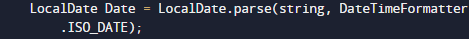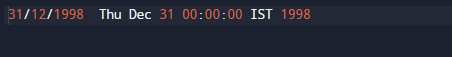# Java Program to Convert String to Date

In this tutorial you will learn about the Java Program to Convert String to Date and its application with practical example.

In this tutorial, we will learn to create a Java program that will Convert String to date   using Java programming.

## Prerequisites

Before starting with this tutorial we assume that you are best aware of the following java programming topics:

• Java Operators.
• Basic Input and Output
• Class and Object.
• Basic Java programming.
• String.
• User define Function.

## How to convert String to Date in Java?

In this tutorial we will learn is “How to convert String value to Date value” using simple code changes and techniques. The best way is to convert String to Date in java using parse() method.

## Java Program to Convert String to Date

In this program we will convert value of “String to Date” . We would first declared and initialized the required variables. Next, we would use inbuilt function to do our task. let have a look at Code.

## OuptutIn above program, we have used the inbuilt format that takes value in string format and returns the value in date format like this “2021-07-13” and returns 2021-07-13.Java Date-Time API that gives as a  parse() methods that convert the String given value into the Date-Time value format.

## Another way to  Convert String to Date

Let us see the  code to convert String to Date in java.

## OutputSo in this tutorial we have learn how to create a program of java that convert  the value is in string format to date format.
For that we use two different approaches to convert the value of string format to string.As shown in above .that its for this tutorial thank you for watching this blog..

In this tutorial we have learn about the Java Program to Convert String to Date and its application with practical example. I hope you will like this tutorial.